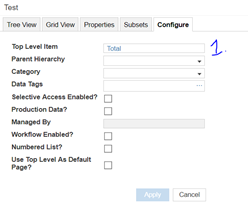# Calcuation of subsets as my allocation basisHi guys, how can i get the totals of my subsets in my formula so the totals of my denominator will only be the totals of the subsets? Thanks in advance.

Tagged:

•Hi,

You can use Sum & Select function to get the value of the total of subset in formula by selecting the Top level item of the list .

Please find the detailed snapshots below

Step 1: Create Top level item in the List.Step 2: Check with the List and the subsetStep 3: In the Module Create a Boolean formatted line Item where the subset is in “Applies to” with TRUE.  As shown belowRefer this line item to get the totals of Subset as in “ss total” line item using Sum & select item.

You will get the desired subset totals in line items.Hope this helps.

Thanks